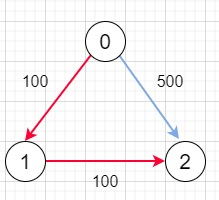Cheapest Flights Within K Stops in C++

C++Server Side ProgrammingProgramming

Suppose we have n cities connected by m flights. Each flight starts from u and arrives at v with a price w. If we have all the cities and flights, together with starting city src and the destination dst, here our task is to find the cheapest price from src to dst with up to k stops. If there is no such route, then return -1.

So, if the input is like n = 3, edges = [[0,1,100],[1,2,100],[0,2,500]], src = 0, dst = 2, k = 1, then the output will be 200To solve this, we will follow these steps −

• Create one data structure called Data, this can hold node, dist and val

• Define one 2D array cost

• cost := one 2D array of order (n + 1) x (K + 10) fill this with infinity

• Define one 3D array graph

• for initialize i := 0, when i < size of flights, update (increase i by 1), do −

• u := flights[i, 0]

• v := flights[i, 1]

• insert { v, flights[i, 2] } at the end of graph[u]

• define one priority queue q

• insert Data(src, 0, 0) into q

• cost[src, 0] := 0

• ans := -1

• while (not q is empty), do −

• temp := top element of q

• curr := temp.node

• delete element from q

• dist := temp.dist

• if curr is same as dst, then −

• return temp.cost

• (increase dist by 1)

• if dist > K + 1, then −

• for initialize i := 0, when i < size of graph[curr], update (increase i by 1), do −

• neighbour := graph[curr, i, 0]

• if cost[neighbour, dist] > cost[curr, dist - 1] + graph[curr, i, 1], then −

• cost[neighbour, dist] := cost[curr, dist - 1] + graph[curr, i, 1]

• insert Data(neighbour, dist, cost[neighbour, dist]) into q

• return -1

Example

Let us see the following implementation to get better understanding −

Live Demo

#include <bits/stdc++.h>
using namespace std;
struct Data{
int node, dist, cost;
Data(int a, int b, int c){
node = a;
dist = b;
cost = c;
}
};
struct Comparator{
bool operator() (Data a, Data b) {
return !(a.cost < b.cost);
}
};
class Solution {
public:
vector<vector<int>> cost;
int findCheapestPrice(int n, vector<vector<int>>& flights, int src, int dst, int K) {
cost = vector<vector<int> >(n + 1, vector<int>(K + 10, INT_MAX));
vector<vector<int> > graph[n];
for (int i = 0; i < flights.size(); i++) {
int u = flights[i];
int v = flights[i];
graph[u].push_back({ v, flights[i] });
}
priority_queue<Data, vector<Data>, Comparator> q;
q.push(Data(src, 0, 0));
cost[src] = 0;
int ans = -1;
while (!q.empty()) {
Data temp = q.top();
int curr = temp.node;
q.pop();
int dist = temp.dist;
if (curr == dst)
return temp.cost;
dist++;
if (dist > K + 1)
continue;
for (int i = 0; i < graph[curr].size(); i++) {
int neighbour = graph[curr][i];
if (cost[neighbour][dist] > cost[curr][dist - 1] + graph[curr][i]) {
cost[neighbour][dist] = cost[curr][dist - 1] + graph[curr][i];
q.push(Data(neighbour, dist, cost[neighbour][dist]));
}
}
}
return -1;
}
};
main(){
Solution ob;
vector<vector<int>> v = {{0,1,100},{1,2,100},{0,2,500}};
cout << (ob.findCheapestPrice(3, v, 0, 2, 1));
}

Input

3, {{0,1,100},{1,2,100},{0,2,500}}, 0, 2, 1

200